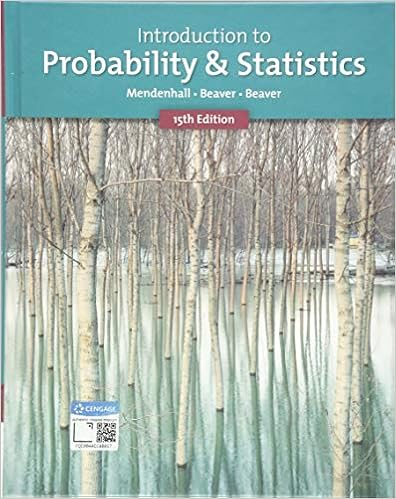# 11 the lengths of pregnancies are normally

• Test Prep
• AjaniG
• 2
• 100% (2) 2 out of 2 people found this document helpful

This preview shows page 1 - 2 out of 2 pages.

##### We have textbook solutions for you!
The document you are viewing contains questions related to this textbook.The document you are viewing contains questions related to this textbook.
Chapter 2 / Exercise 32
Introduction to Probability and Statistics
Beaver/MendenhallExpert Verified
11. The lengths of pregnancies are normally distributed with a mean of 268 and a standard deviation of 15 days. In a letter to Dear Abby, a wife claimed to have given birth 308 days after a brief visit from her husband who was serving in the Navy. Find the probability of a pregnancy lasting 308 days. Is this unusual?
##### We have textbook solutions for you!
The document you are viewing contains questions related to this textbook.The document you are viewing contains questions related to this textbook.
Chapter 2 / Exercise 32
Introduction to Probability and Statistics
Beaver/MendenhallExpert Verified
Statistics Exam 3 review p.2 12. The random number generator on the TI 83 calculator yields numbers from a uniform distribution of values between 0 and 1 with a mean of 0.500 and a standard deviation of 0.289 . If 100 random numbers are generated, find the probability that their mean is less than .57? 13. The amount of nicotine in a brand of cigarettes have a mean of 0.941 g and a standard deviation of 0.313g . Find the probability of randomly selecting 40 cigarettes with a mean of .882 grams or less. SOLUTIONS
0.5497
7.2: Confidence Interval ρ (z score)and find n 7.3: C.I. μ (z score) and find n 1. In a recent study 85 people said that they were satisfied with their present home and 25 people said they were not. Find the 90% confidence interval of the true proportion of individuals who are satisfied with their present home. 2. The average weight of 60 randomly selected compact automobiles was 2627 pounds. The population standard deviation was 400 pounds. Find the 99% confidence interval of a true mean weight of the automobiles. 3. A U.S. Travel Data Center's survey of 1500 adults found that 42% of respondents stated that they favor historical sites as vacations. Find the 95% confidence interval of the true proportion of all adults who favor visiting historical sites as vacations. 4. You want to estimate the percentage of US statistics students who get grades of B or higher. How many such students must you survey if you want 97% confidence that the sample percentage is off by no more than two percentage points? Solutions
•••